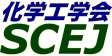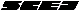## \$B^5-G09V1i(B

\$B:G=*99?7F|;~!'(B2009-03-11 14:01:08

### \$B3X2q>^(B

 \$BBh(B 3 \$BF|!&(BP \$B2q>l(B P304 \$B!V%J%NN3;R:`NA\$N9g@.\$*\$h\$S5!G=2=5;=Q\$N?7E83+!W(B (10:00\$B!A(B10:40) (\$B9-Bg(B) \$B1|;3(B \$B4n5WIW(B \$B;a(B P306 \$B!VE}9g2=5;=Q\$N3+H/\$H\$=\$N1~MQ\$K4X\$9\$k8&5f!W(B (10:40\$B!A(B11:20) (\$BEl9)Bg(B) \$BCg(B \$BM&<#(B \$B;a(B

### \$B8&5f>^(B

 \$BBh(B 3 \$BF|!&(BP \$B2q>l(B P308 \$B!V5\$Aj= (11:20\$B!A(B12:00) (\$B1'ET5\Bg(B) \$B0KF#(B \$BD> P313 \$B!V?75,%^%\$%/%m%j%"%/%?!<\$N3+H/\$H\$=\$l\$rMQ\$\$\$?H?1~@)8fK!\$K4X\$9\$k8&5f!W(B (13:00\$B!A(B13:40) (\$B5~Bg(B) \$BA0(B \$B0lW"(B \$B;a(B P315 \$B!VB?9&9=B\$Kl\$NHy:Y9=B\$@)8f\$K4X\$9\$kKl9)3XE*8&5f!W(B (13:40\$B!A(B14:20) (\$B?@8MBg(B) \$B>>;3(B \$B=(?M(B \$B;a(B

### \$B8&5f>)Ne>^(B

 \$BBh(B 3 \$BF|!&(BP \$B2q>l(B P317 \$B!V%+%j%C%/%9%"%l!<%sM6F3BN\$K\$h\$k%?%s%Q%/ (14:20\$B!A(B14:40) (\$B5\:jBg(B) \$BBgEg(B \$BC#Li(B \$B;a(B P318 \$B!V (14:40\$B!A(B15:00) (\$BElBg(B) \$B2O86(B \$B@59@(B \$B;a(B P319 \$B!V;0 (15:00\$B!A(B15:20) (\$B6eBg(B) \$B8E;3(B \$BDL5W(B \$B;a(B P320 \$B!V%j%A%&%`Fs (15:20\$B!A(B15:40) (\$B;37ABg(B) \$B>>ED(B \$B7=8g(B \$B;a(B P321 \$B!VD94|5!G=0];}\$r (15:40\$B!A(B16:00) (\$B6eBg(B) \$B?eK\(B \$BGn(B \$B;a(B

### \$B5;=Q>^(B

 \$BBh(B 2 \$BF|!&(BXA \$B2q>l(B XA202 \$B!V5[Ce5;=Q\$rMQ\$\$\$?%P%\$%*%,%9M-8zMxMQ%7%9%F%`3+H/!W(B (9:20\$B!A(B9:40) (\$BBg:e%,%9(B) \$B4X(B \$B7z;J(B \$B;a(B\$B!&(B(\$BBg:e%,%9%(%s%8%K%"%j%s%0(B) \$B>(B \$B9'I'(B \$B;a(B XA203 \$B!V0!NW3&?e\$r1~MQ\$7\$?3&LL3h@-:^\$N9g@.%W%m%;%9!W(B (9:40\$B!A(B10:00) (\$B%U%!%F%#%1%_%+%k(B) \$BEDC<(B \$B=\$(B \$B;a(B\$B!&(B(\$B2V2&(B) \$BC*66(B \$B??0lO:(B \$B;a(B\$B!&(B\$BGrBt(B \$BIp(B \$B;a(B\$B!&(B\$B1'Ln(B \$BK~(B \$B;a(B\$B!&(B\$Bc7F#(B \$BL@NI(B \$B;a(B XA204 \$B!V%U%CAG7OCO5e29CH2=%,%9\$NJ,2r=hM}AuCV\$N3+H/!W(B (10:00\$B!A(B10:20) (\$B;:Am8&(B) \$B!I'(B \$B;a(B\$B!&(B(\$B1A86@=:n=j(B) \$BBgN\$(B \$B2m><(B \$B;a(B\$B!&(B\$B?9(B \$BMN0l(B \$B;a(B\$B!&(B(\$B:4@\$J]9b@l(B) \$BD9ED(B \$B=(IW(B \$B;a(B\$B!&(B(\$B6eBg(B) \$B4_ED(B \$B>;9@(B \$B;a(B XA205 \$B!V?75,1v;@;@2=%W%m%;%9\$N3+H/\$H9)6H2=!W(B (10:20\$B!A(B10:40) (\$B=;M'2=3X(B) \$B?9(B \$B9/I'(B \$B;a(B\$B!&(B\$B0\$It@n(B \$B90L@(B \$B;a(B\$B!&(B\$B4X(B \$B9RJ?(B \$B;a(B\$B!&(B\$BNkED(B \$BE/Li(B \$B;a(B\$B!&(B(\$B=;M'%1%_%+%k%(%s%8%K%"%j%s%0(B) \$B0\$It(B \$BCi(B \$B;a(B XA206 \$B!V(BPCB\$BL5322==hM}\$r (10:40\$B!A(B11:00) (\$B;0I)=E9)6H(B) \$BLn:j(B \$B><9((B \$B;a(B\$B!&(B\$BDM86(B \$B@i9,?M(B \$B;a(B\$B!&(B\$B_7DE66(B \$BE0:H(B \$B;a(B\$B!&(B\$BEZ66(B \$B?8:n(B \$B;a(B\$B!&(B\$BN686(B \$B7i(B \$B;a(B

### \$B5;=Q>)Ne>^(B

 \$BBh(B 2 \$BF|!&(BXA \$B2q>l(B XA207 \$B!VFqMO2r@-%j%s;@1v\$N>=@O8=>]\$rMxMQ\$7\$?2 (11:00\$B!A(B11:20) (\$B1A86@=:n=j(B) \$BEgB<(B \$BOB>4(B \$B;a(B XA208 \$B!V%^%\$%/%m%A%c%s%M%k\$rMQ\$\$\$?EE;R%Z!<%Q! (11:20\$B!A(B11:40) (\$BAn8&2=3X(B) \$B9b66(B \$B9'FA(B \$B;a(B XA209 \$B!V%7%j%+7OB?9& (11:40\$B!A(B12:00) (\$B%N%j%?%1%+%s%Q%K!<%j%_%F%I(B) \$B5HLn(B \$BBY(B \$B;a(B

\$B9V1i%W%m%0%i%`(B
\$B2=3X9)3X2q(B \$BBh(B74\$BG/2q(B(\$B2#IM(B)(C) 2009 (\$B
Most recent update: 2009-03-11 14:01:08
E-mail: inquiry-74awww2.scej.org
This page was generated byeasp 2.26; proghtml 2.26b (C)1999-2008 kawase